# Presentation-Ready Summary Tables with gtsummary

We are thrilled to introduce you to the gtsummary package!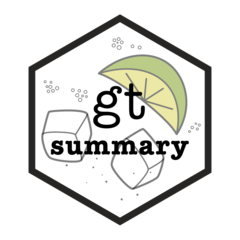The gtsummary package provides an elegant and flexible way to create publication-ready analytical and summary tables in R.

The motivation behind the package stems from our work as statisticians, where every day we summarize datasets and regression models in R, share these results with collaborators, and eventually include them in published manuscripts. Many of our colleagues had our own scripts to create the tables we needed, and even then would often need to modify the formatting in a document editor later, which did not lead to reproducible results.

At the time we created the package, we had several ideas in mind for our ideal table summary package. We also wanted our tables to be able to take advantage of all the features in RStudio’s newly released gt package, which offers a variety of table customization options like spanning column headers, table footnotes, stubhead label, row group labels and more. So, gtsummary was born!

Here’s what you can do with gtsummary:

• Summarize data frames or tibbles to present descriptive statistics, compare group demographics (e.g creating a Table 1 for medical journals), and more!
• Summarize regression models. Using `broom::tidy()` in the background, gtsummary plays nicely with many model types (lm, glm, coxph, glmer etc.). You may also use custom functions to summarize regression models that do not currently have broom tidiers.
• Customize gtsummary tables using a growing list of formatting/styling functions: everything from which statistics and tests to use to how many decimal places to round to, bolding labels, indenting categories and more!
• Report statistics inline from summary tables and regression summary tables in R markdown. Make your reports completely reproducible!
• Leverage compatibility with multiple R Markdown outputs to create beautiful, reproducible reports in a variety of formats (HTML, PDF, Word, RTF)

Install gtsummary from CRAN with the following code:

``````install.packages("gtsummary")
``````

## Summarize descriptive statistics

Throughout the post we will use an example dataset of 200 subjects treated with either Drug A or Drug B, with a mix of categorical, dichotomous, and continuous demographic and response data. The dataset has label attributes (using the labelled package) for column names.

``````sm_trial <- trial %>% select(trt, age, response, grade)

#> # A tibble: 6 x 4
#>   <chr>  <dbl>    <int> <fct>
#> 1 Drug A    23        0 II
#> 2 Drug B     9        1 I
#> 3 Drug A    31        0 II
#> 4 Drug A    NA        1 III
#> 5 Drug A    51        1 III
#> 6 Drug B    39        0 I
``````

In one line of code we can summarize the overall demographics of the dataset!

Notice some nice default behaviors:
💜 Detects variable types of input data and calculates descriptive statistics
💜 Variables coded as 0/1, TRUE/FALSE, and Yes/No are presented dichotomously
💜 Recognizes `NA` values as “missing” and lists them as unknown
💜 Label attributes automatically printed
💜 Variable levels indented and footnotes added

``````tbl_summary_1 <- tbl_summary(sm_trial)
``````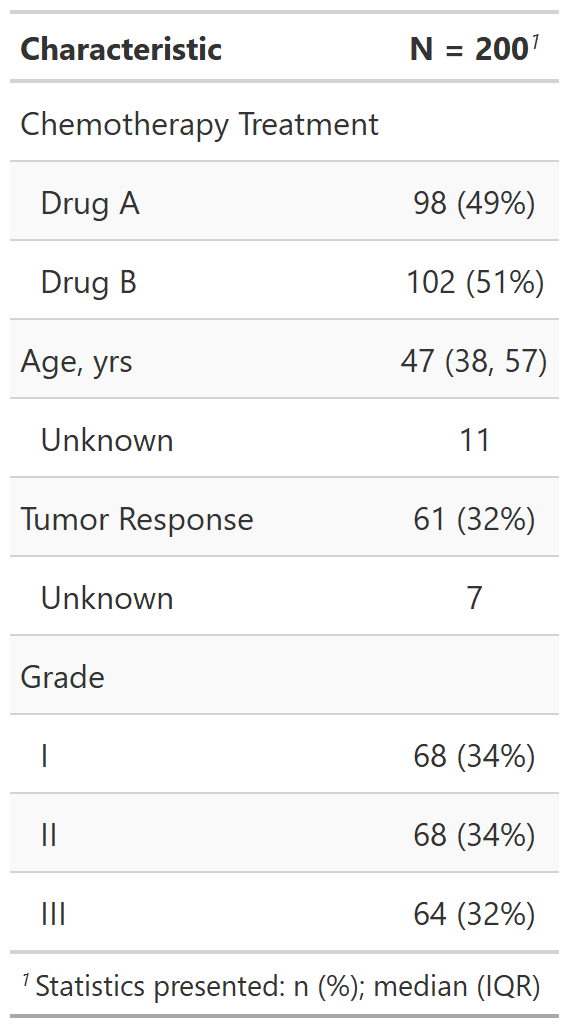Start customizing by adding arguments and functions

Next you can start to customize the table by using arguments of the `tbl_summary()` function, as well as pipe the table through additional gtsummary functions to add more information, like p-value to compare across groups and overall demographic column.

``````tbl_summary_2 <- sm_trial %>%
tbl_summary(by = trt) %>%
bold_labels()
``````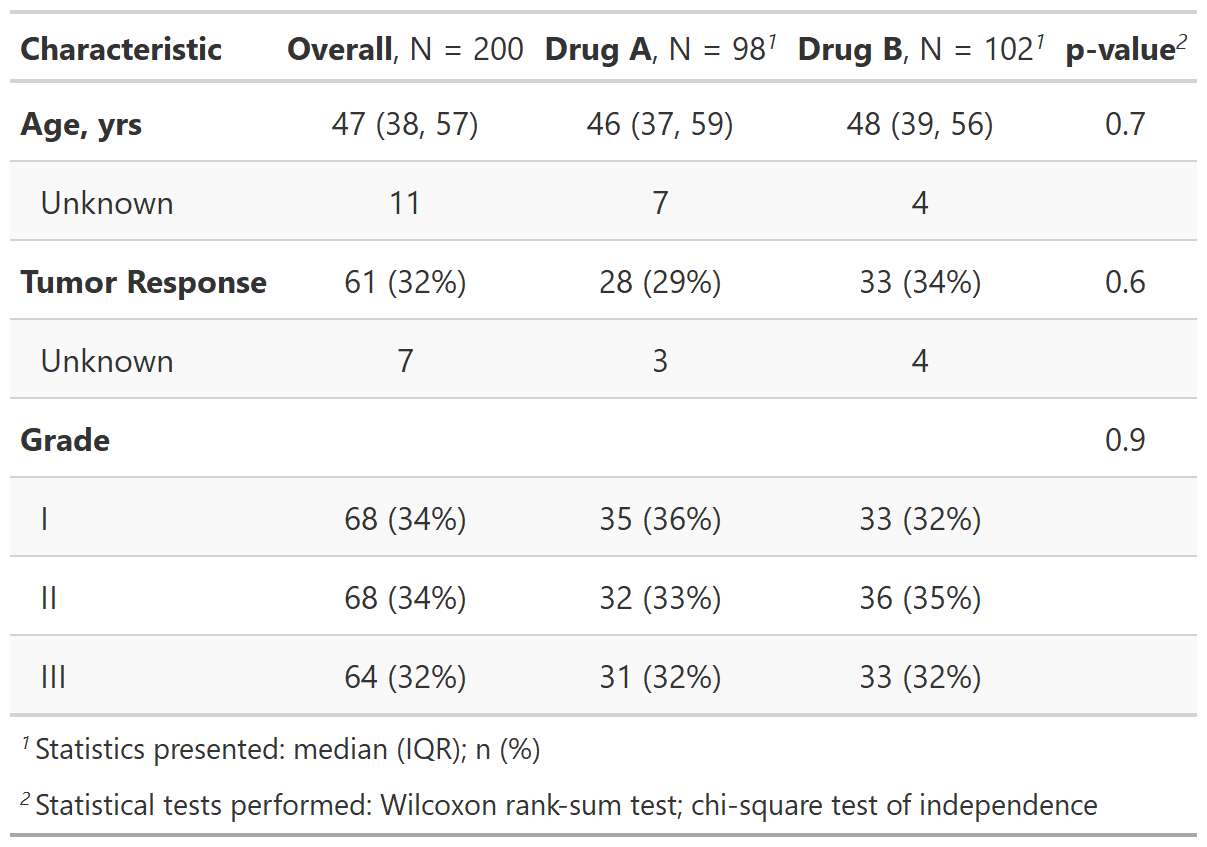Customize further using formula syntax and tidy selectors

Most arguments to `tbl_summary()` and `tbl_regression()` require formula syntax:

select variables ~ specify what you want to do

• To select, use quoted or unquoted variables, or minus sign to negate (e.g. `age` or `"age"` to select, `-age` to deselect)
• Or use any {tidyselect} functions, e.g. `contains("stage") ~ ...`, including type selectors
• To specify what you want to do, some arguments use {glue} syntax where whatever is in the curly brackets gets evaluated and passed directly into the string. e.g `statistic = ... ~ "{mean} ({sd})"`
``````tbl_summary_3 <- sm_trial %>%
tbl_summary(
by = trt,
statistic = list(
all_continuous() ~ "{mean} ({sd})",
all_categorical() ~ "{n} / {N} ({p}%)"),
label = age ~ "Patient Age") %>%
pvalue_fun = function(x) style_pvalue(x, digits = 2))
``````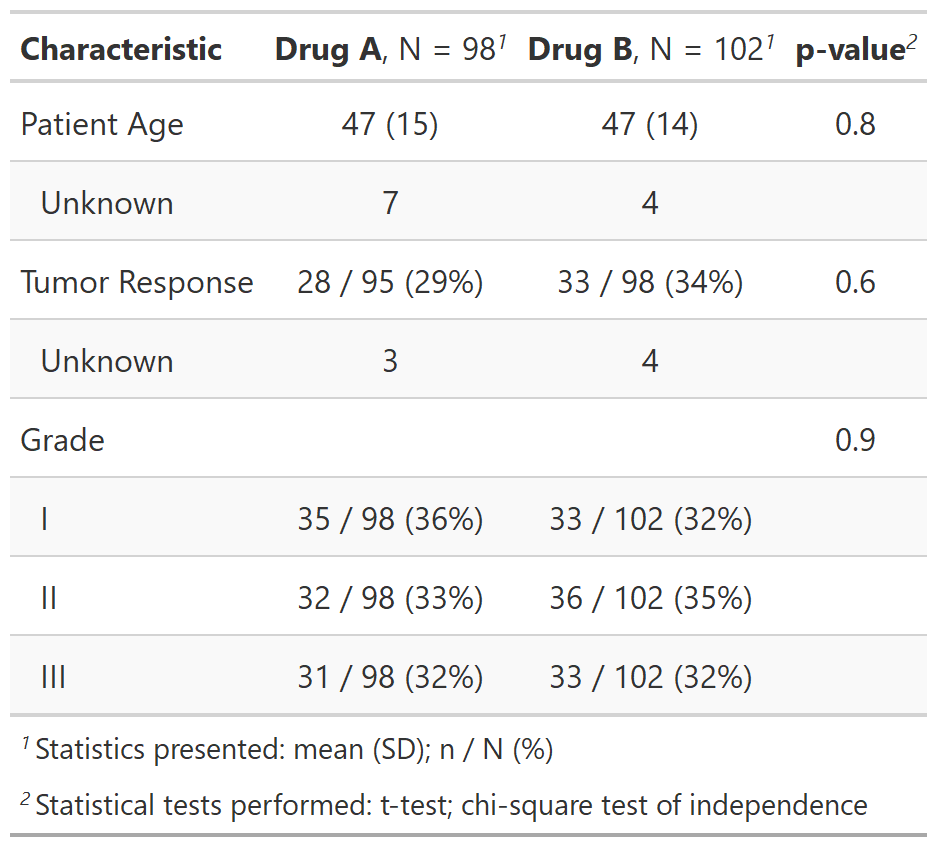## Summarize regression models

First, create a logistic regression model to use in examples.

``````m1 <- glm(response ~ trt + grade + age,
data = trial,
family = binomial)
``````

`tbl_regression()` accepts regression model object as input. Uses {broom} in the background, outputs table with nice defaults:

💜 Reference groups added to the table
💜 Sensible default number rounding and formatting
💜 Label attributes printed

``````tbl_reg_1 <- tbl_regression(m1, exponentiate = TRUE)
``````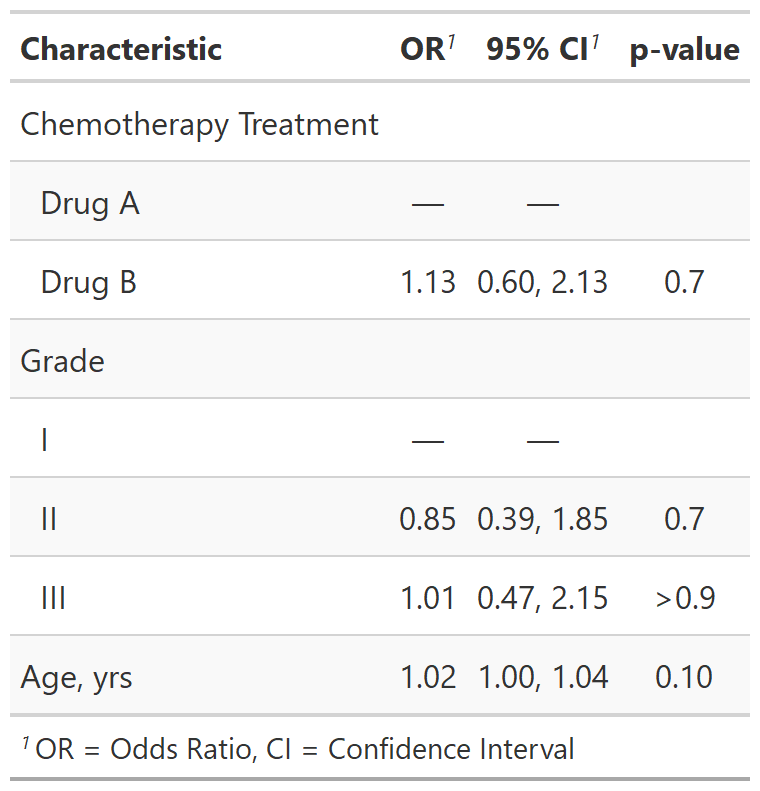We have a growing list of vetted models that can be passed to `tbl_regression()`. You may also pass a custom tidier for model types that are not yet officially supported!

## Join two or more tables

Oftentimes we must present results for multiple outcomes of interest, and there are many other reasons you might want to join two summary tables together. We’ve got you covered!

In this example we can use `tbl_merge()` to merge two gtsummary objects side-by-side. There is also a `tbl_stack()` function to place tables on top of each other.

``````library(survival)

tbl_reg_3 <-
coxph(Surv(ttdeath, death) ~ trt + grade + age,
data = trial) %>%
tbl_regression(exponentiate = TRUE)

tbl_reg_4 <-
tbl_merge(
tbls = list(tbl_reg_1, tbl_reg_3),
tab_spanner = c("**Tumor Response**", "**Time to Death**")
)
``````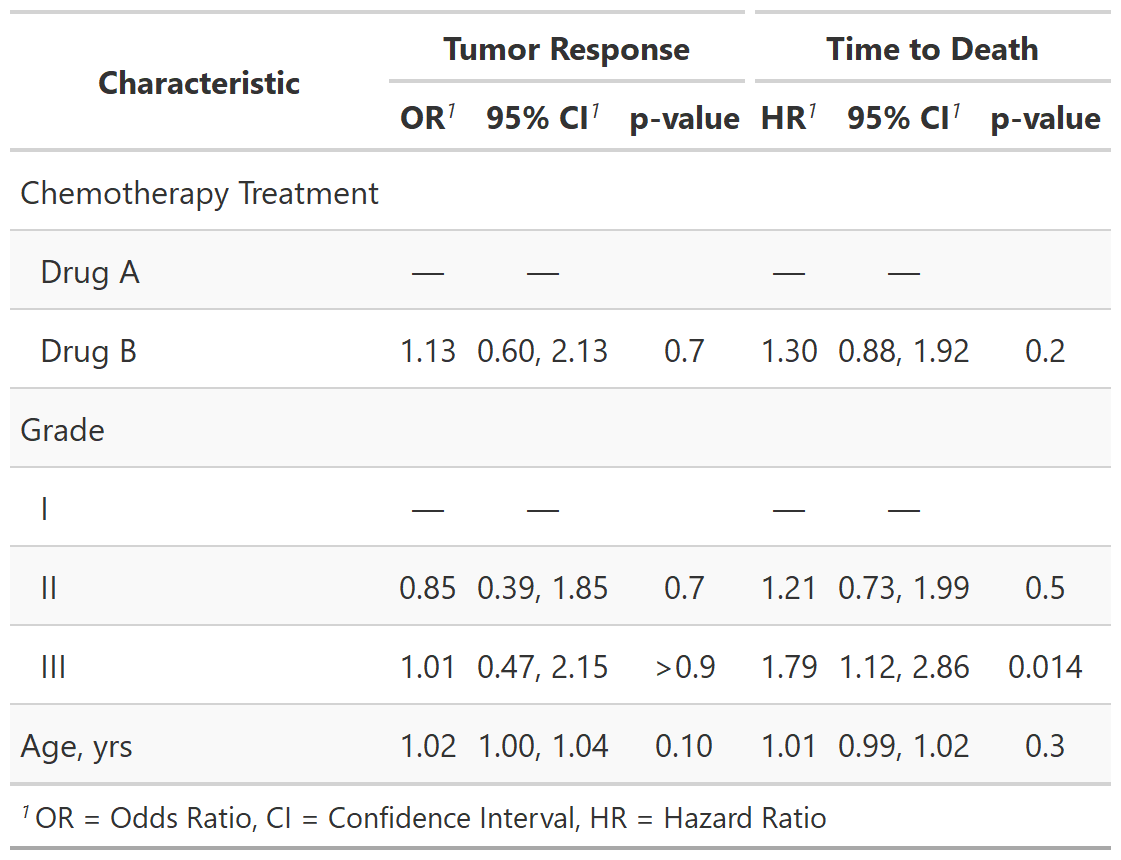## Report results inline

Tables are important, but we often need to report results in-line in a report. Any statistic reported in a gtsummary table can be extracted and reported in-line in a R Markdown document with the `inline_text()` function.

`inline_text(tbl_reg_1, variable = trt, level = "Drug B")`

1.13 (95% CI 0.60, 2.13; p=0.7)

• The pattern of what is reported can be modified with the `pattern = ` argument.

• Default is `pattern = "{estimate} ({conf.level*100}% CI {conf.low}, {conf.high}; {p.value})"`.

## gtsummary + R Markdown

The gtsummary package was written to be a companion to the gt package from RStudio. But not all output types are supported by the gt package (yet!). Therefore, we have made it possible to print gtsummary tables with various engines.

Review the gtsummary + R Markdown vignette for details.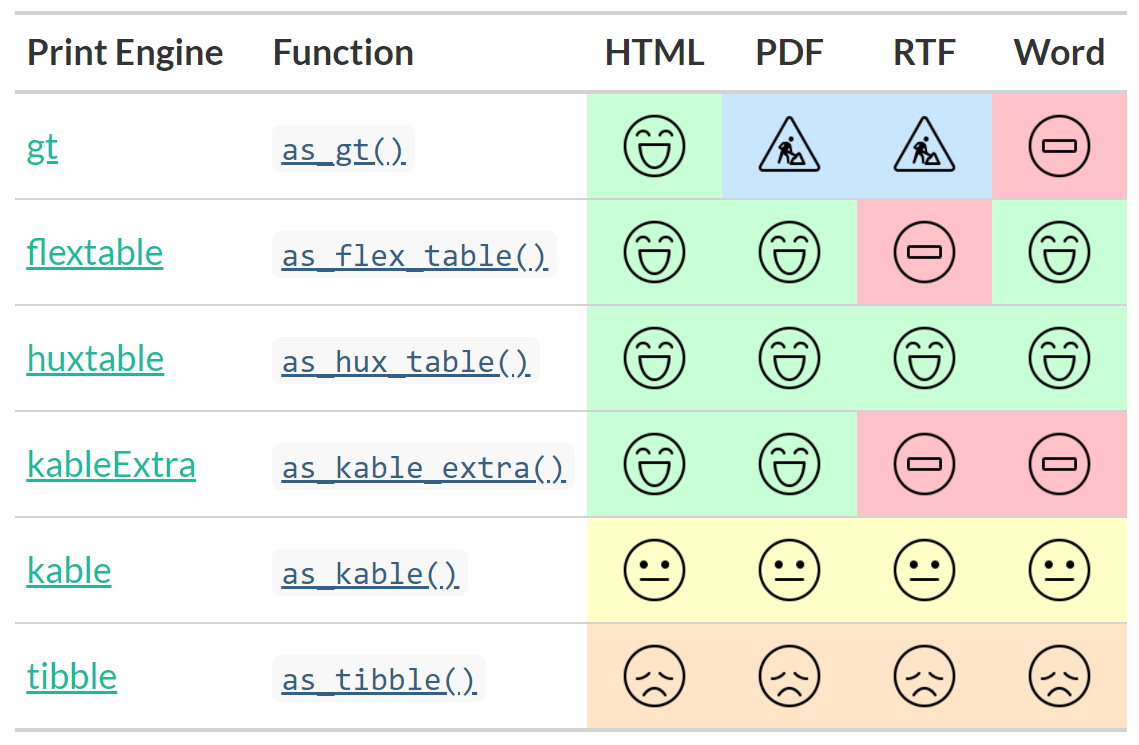## Using external customization functions

It’s natural a gtsummary package user would want to customize the aesthetics of the table with some of the many functions available in the print engines listed above.

Once you convert a gtsummary object to another kind of object (e.g. gt), every function compatible that object will be available to use!

Example workflow and code using gt customization:

1. Create a gtsummary table.
2. Convert the table to a gt object with the `as_gt()` function. (Also available: `as_flextable()`, `as_kable_extra()`
3. Continue formatting as a gt table with any gt function.
``````tbl_summary_5 <- sm_trial %>%
# create a gtsummary table
tbl_summary(by = trt) %>%
# convert from gtsummary object to gt object
as_gt() %>%
# modify with gt functions
gt::tab_spanner(
label = "Randomization Group",
columns = starts_with("stat_")
) %>%
gt::tab_options(
table.font.size = "small",
``````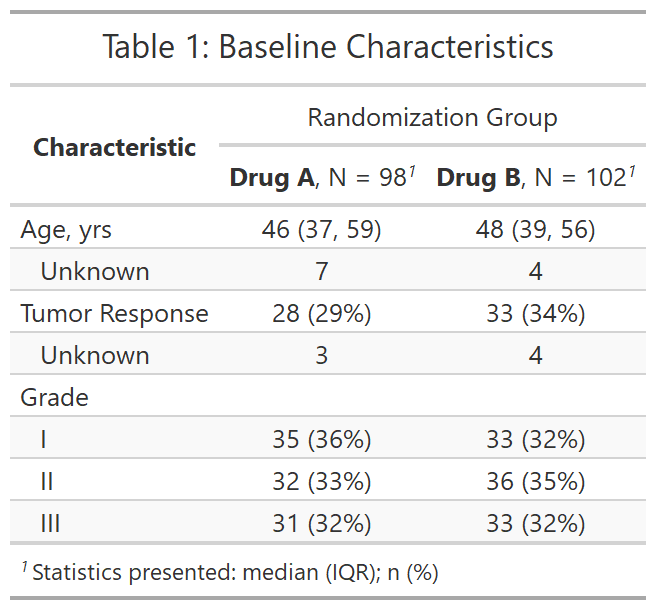There are a few other functions we’d like you to know about!

See the full list of gtsummary functions here. You can use them to do all sorts of things to your tables, like:

• Use custom functions for calculating p-values and reporting any statistic for continuous variables (including user-written functions)
• Bold and italicize labels and levels
• Present missing data in various ways
• Sort variables by significance (`sort_p()`); sort categorical variables by frequency
• Calculate cell percents and row percents (default is column-wide)
• Report p-values for select variables (`add_p(include = ...)`); report q-values (like false discovery rate)
• Set global rounding options with themes (for estimates, confidence intervals, and p-values)

There is a growing gallery of tables which highlights some of the many customization options!

## Wrap-up

The gtsummary package website contains well-documented functions, detailed tutorials, and examples!

If you have any questions on usage, please post to StackOverflow and use the gtsummary tag. We try to answer questions ASAP! You can also report bugs or make feature requests by submitting an issue on GitHub.